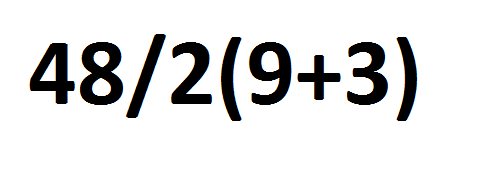}

# Why Order Matters

6/19/2019

While it may seem strange, sometimes it is possible for a mathematical expression to have two different right answers. Consider:This expression has been discussed on the Internet since 2011! The correct answer could be either 2 or 288. Really. It depends on how the expression is evaluated. To understand that we have to understand that expressions are generally evaluated left right when the operators have the same precedence. That is some operations such as multiply and divide happen before addition or subtraction.

In the expression above, however, there is some ambiguity: that "2" in front of the "(9+3)" is a bit special sometimes. It is called "multiplication by juxtaposition". That is, there is no "x" as in 3 x 5., but it still means multiply. In many circles, it takes makes the multiplication higher precedence than the divide. That means it happens before the usual left-to-right rule. In other circles, the divide happens first. This is based on the "order of evaluation".

If the divide is first, we can rewrite the expression as (48/2) x (9+3) which is 24 x 12 or 288. If the multiplication comes first, we get 48 /( 2 x (9+3)) which is 48/24 giving 2. That is a significant difference.

Programming languages designed to do mathematics (as many of them are) have to have rules so there can be no ambiguity. In a programming language using the rules of C (which can be found at https://en.wikipedia.org/wiki/Order_of_operations) the two mathematical interpretations of the expression could be written as: (48/2) * (9+3) or (48/(2*(9+3)), much like the above. The common 48 / 2 * (9 + 3) would evaluate left to right giving the (48/2) x (9+3) result. An experienced programmer would know that and have no problem with interpretation. But it isn't always so simple.

In C there are 15 levels of precedence. One can easily write code using the proper order that another programmer could misinterpret due to lack of experience or lack of attention when reading the code. It is a good idea, therefore, to use parenthesis (the highest precedence in both mathematics and programming languages) to disambiguate any complex expressions so that those looking at code interpret it as intended. It also helps writing it correctly: sometimes even experienced programmers err in getting complex expressions correct.

It is important to note that some programming languages have no notion of precedence of operators and evaluate strictly left to right or right to left while some languages have further complications.

These issues come to a head when translating a mathematical expression into an expression in a programming language. The programmer must know what the writer of the mathematical expression intended. In the example with which we started, does the juxtaposition multiplication have higher precedence or not? This may require researching the policy of a publication in which the expression occurs or the background of the writer.

In the future, we'll look at different ways of writing expressions in a few unusual programming languages including those that put the operation first "add 5 to 3" and last "3 5 +".### Written by John McDermott

John McDermott, CPTD, started his work in computer security in 1981 when he caught an intruder in a system he was managing. In recent years his consulting has included security consulting for small businesses. He is Security+ and CCP certified. In his 30 years with Learning Tree John has written and taught courses in programming, networking and computer security. He is the co-author of Learning Tree’s course System and Network Security: A Comprehensive Introduction. John is currently a learning and development consultant in northern New Mexico. He lives in a house made of earth with his wife, who is an artist.

Chat With Us